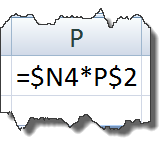# Referring to Ranges in Formulas for your Excel Dashboard TemplatesThere are many ways to save time using Absolute and Relative References in Templates created in Excel Dashboards

When building Excel Dashboard Templates, you will frequently refer to cells or ranges in formulas.  After which, you will typically copy those formulas to other like data points that are doing similar calculations.  So you should know the ins and outs of Cell References in order to save time and build powerful formulas.

There are 2 types of References and 4 ways to use them.  Let me show you how you can use these in your
Dashboard Templates.

The easiest way to think of a cell/range reference is by thinking of how is this cell/range being referred?  Should Excel refer to the cell relative to my current position? Or should Excel refer to it absolutely in the same row/column/cell from my current position.

A Relative References

Anytime you create a formula in Excel with a reference to another cell or range of cells, the default is a relative reference to that cell or range.  Remember that a relative reference to a cell or range is relative to the cell in which the formula is located.  This is a great way to create a
formula that you wish to reuse in other cells where the data shifts to another cell or range.  A good example for your Sales Dashboard Template is a Grand Total row that sums how much profit you have made by product for the month of January, and you want to reuse the formula for
the months of February to December.

For instance, if you are summing total income in cell B8 with this formula:    =SUM(B2:B7)  you are asking Excel to Sum the 6 cells above
you.  If you copy that formula one column over, you are asking Excel to Sum the 6 cells above C8.  If you copy the formula down to cell G51, you are asking excel to Sum the 6 cells above G51.  Excel doesn’t remember or care that you originally summed the cells B2 thru B7, it just knows that you want to sum the 6 cells above the RELATIVE position of the formula you entered.

Absolute References

An absolute reference to a cell or range of cells is not the default but can be very useful when creating your Excel Dashboard Template.  An absolute reference tells Excel to ALWAYS refer to this one cell location or range.  So that means that when you copy a formula to another cell it will not change the absolute references of the formula.  A good example of the uses of this feature would be when you want to refer to a constant.

For instance, if you want to apply a tax percentage to your monthly gross profits it would be best to put this percentage in a cell that can be
changed versus hard-coding it in each month.  Therefore you can create the formula once and using an Absolute Reference to the cell with the tax percentage, you can copy the formula to all the months and all of the formulas will reference the same tax percentage cell.

Mixed Relative and Absolute References.

A mixed cell/range reference can also be very powerful when copying formulas across columns or rows so you need to know how to use this
powerful technique.  A mixed cell reference holds one part of the cell reference Absolute and the other part Relative when referenced in other cells and formulas.  For instance, if you want your formula to keep a cell’s column absolute you would reference that cell with a \$ before the
column letter (i.e. \$a1).  This means that wherever you copy the formula to another cell, it will always look at the “A” column for the current row that you place the formula.  Likewise, if you want to make sure and always reference the same row for your formula regardless of the column that you copy it to, you will want to put a “\$” in front of the row number of your cell reference (i.e. a\$1).  Why would you want to do this type of reference?  Perhaps you have a formula in one column and you want to copy across multiple columns to calculate comparison data without retyping the same similar formula in each adjacent column.  For instance, what if you were thinking of moving your business to another city and you want to calculate how much you would have to pay in taxes per city per product type.  You can create one formula in the upper left area of your final data table and if structured right you can simply copy the formula down ONCE to all rows and columns and save lots of time and reduce errors.  See the example below:

Now you can see the power of knowing Absolute and Relative References when creating your own Excel Dashboard Templates.